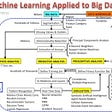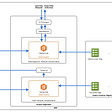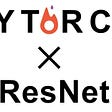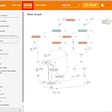# Tips and Tricks for advancing Tensorflow API: Subclassing, Data processing, Extensions

## Tensorflow

The TensorFlow framework provides many useful classes and features for an end-to-end machine learning pipeline. Most aspects of the provided functions from reading data to training logic are highly customizable. We will explore many functionalities of Tensorflow to enable the implementation of complex deep learning algorithms or accelerate the development process of deep learning applications, focusing on image data and CNNs.

This post will not be an in-depth explanation of certain topics but rather a general introduction of low-level TF and useful TF classes, while I will link references to the documentation and helpful resources for further information and examples. I aim to introduce my techniques for using TensorFlow when developing machine learning projects. Feel free to discuss better ways to exploit TensorFlow for training models through comments.

The aspects of Tensorflow we will discuss in this post are:

• tf.data.Dataset
• preprocessing data
• data augmentation(ImageDataGenerator, tf.keras.preprocessing.image)
• tensorflow_datasets(tfds)
• custom training loop
• overriding tf.keras.Model, subclassing of TensorFlow objects
• TensorFlow extensions(tf_a, tfg, more)

## tf.data.Dataset object [docs]

Represents a potentially large set of elements.

tf.data.Dataset is an API provided by TensorFlow for implementing input pipelines. The process of loading, preprocessing, augmenting, and iterating on data are in the scope of the API. The full procedure is designed to operate on a `tf.data.Dataset` object.

We can create `tf.data.Dataset` objects simply by mapping a list, as in the snippet below. In the first example, we can simply iterate through the dataset in order using for-loops.

There are also very convenient methods of the dataset such as the `shuffle` and `batch` the operation described in the second example. The operations literally shuffle the dataset and make batches from the dataset. The `repeat(n)` operation repeats the dataset n times, while when n is undefined the dataset is repeated infinitely.

Sometimes datasets can be defined as more complex structures. One common form of data is data in a pair of (image, label). Such data can be defined using dictionaries as in the example above. We can also see that the images and labels are grouped after the shuffle operation.

We can also construct datasets with 3+ components or use other functions instead of `tf.data.Dataset.from_tensor_slices`, which is further described in the documentation.

One final method `tf.data.Dataset` to cover is the `dataset.map(map_f)` method, which applies some `map_f` method for all the elements in the dataset individually. In the example above, the `example_map` function is applied to add 5 to all the label values.

The first part of every machine learning application is reading data. You could simply read data using other libraries (e.g. NumPy, PIL, OpenCV …) and store them in a NumPy array(or a simple array). One downside of actually reading and storing all the data in a variable is that it consumes too much RAM and will be infeasible for a large dataset.

TensorFlow provides dynamic approaches to read data, that can be represented as defining a function to read and store a batch of the data dynamically at runtime instead of storing the data directly. Because training is done based on the output of the input pipeline, the function must contain the definition of the preprocessing and augmentation step also. This process is accelerated using multiprocessing.

Procedures for the most general cases are provided by TF, while they also can be fully customizable. Two key functionalities in TF for reading data are using the all-in-one pipeline such as `image_dataset_from_directory` and directly defining a function to create an `tf.data.Dataset` object, which is more customizable.

## image_dataset_from_directory[docs][tutorial]

Generates a `tf.data.Dataset` from image files in a directory.

image_dataset_from_directory is a handy function for reading data in specific directory structures. For example, data in the following structure, which is common in image datasets such as ImageNet, we can read using the following code. We can also make slight modifications for applications to different types of data and also add some basic preprocessing by tweaking the arguments.

`training_data/...class_a/......a_image_1.jpg......a_image_2.jpg...class_b/......b_image_1.jpg......b_image_2.jpg`

Code:

`train_ds = image_dataset_from_directory(    directory='training_data/',    labels='inferred',    label_mode='categorical',    batch_size=32,    image_size=(256, 256))`

## Custom functions + dataset.map [example]

Since I discovered this snippet of a custom implementation to read images from a directory from the Pix2Pix Tensorflow tutorial, I personally mostly use this custom implementation to read data.

The snippet above maps a tf. data.Dataset of paths through the `read_image` function, that reads the images in TensorFlow graph mode. An advantage of this method is that it is very customizable in terms of the directory structure and data format since I often struggled to integrate different forms of the label when using image_dataset_from_directory.

When we define variables such as `train_dataset` , Tensorflow doesn’t always actually read and store all the data in the variable. Instead, a mapping function(Tensorflow graph) is created in train_dataset and whenever we access the values of the dataset using an iterator, the mapping executed and the data is read. Because the variable is virtual, we can’t simply print out values or index the `train_dataset` such as `print(train_dataset) or train_dataset`.

# Data Augmentation

## ImageDataGenerator [docs][tutorial]

Generate batches of tensor image data with real-time data augmentation.

`ImageDataGenerator` provides a tool to implement data augmentation. To use `ImageDataGenerator` for data augmentation, we must first declare an instance containing the augmentation settings from the pool of augmentations below.

Augmentation is applied to data using two methods. First, when the dataset is already loaded or defined as a tf.data.Dataset variable, we can use `datagen.flow(x, y)` to build an augmented dataset as in the code below.

We can also use `datagen.flow_from_dirctory(directory)` to read directly from the files as in the code below. This method is very often used because it is concise while also being relatively well applicable to many datasets.

## Custom Augmentation using tf.image [docs]

You can perform custom data augmentation using functions provided in `tf.image.random_*` and define a tf graph. This can be applied to the data by mapping the augmentation pipeline to the dataset object.

## tf.keras.layers.experimental.preprocessing [docs][tutorial]

Integrate preprocessing into the model using the layer interface.

This module provides data augmentation functions in Keras layers. Therefore, we can represent the augmentation process as a sequential model, or simply add augmentation layers to the beginning of an existing neural network.

For example, the following code will define and perform data augmentation. The layers don’t do anything at test time.

I personally believe this concept of introducing data augmentation as a layer is amazing. We can also define custom data augmentations(e.g. mixup, cut mix) as a custom layer using subclassing, which will be introduced below.

## TFDS(Tensorflow Datasets) [catalog]

One last handy feature provided by TensorFlow is the `tensorflow_datasets`, or the `tfds` library, which provides the possibly most simple way to download and read around 300 major datasets of different formats.

TFDS datasets can be downloaded and read into a tf.data.Dataset object using the above single line of code. To download a dataset using TFDS, you can simply find the key of the dataset, which is the exact name in the TFDS catalog, and call `tfds.load(key)`. The structure of each dataset is described separately on the explanation page.

# Custom Training Loop

The Keras & TF2.0 style programming which many of you might be used to is likely to be utilizing the high-level APIs such as `model.compile` and `model.fit`. Although the default training procedure is highly customizable to a degree, some tasks such as GANs require more complex and custom training loops.

In this case, we can manually implement the process of one training step and repeat the step following the data iterator. The snippet below implements a generic training step of a network. `tf.GradientTape` in line 2 declares a variable `tape` that performs automatic differentiation in its scope. Line 3 and 4 implement the inference procedure and map a connection between the loss value and the network parameters. In line 5, the `tape` variable performs automatic differentiation and returns the gradient of the network weights. Finally, line 6 applies the computed gradients through an optimizer.

The code above is the basis for a custom training step. This might be unnecessary for default CNNs and simple networks, but it is a critical API of TensorFlow for building sophisticated training procedures.

The official TF tutorial of implementing DCGAN in TensorFlow also does a great job of explaining this concept, in a more complex setting.

# Subclassing TF classes

According to Tensorflow, the `tf.Module` class is the most basic module for its implementations. The base of all layers `tf.keras.layers.Layer` inherits from `tf.Module` while the base of all models `tf.keras.Model` inherits from `tf.keras.layers.Layer`.

Just like how every layer in Keras such as `Conv2D` , `MaxPooling2D` are defined, we can override the layer class to make a custom layer. We can also subclass the base model interface and override the default methods for a custom training loop.

## Custom layers: tf.keras.layers.Layer [docs]

This is the class from which all layers inherit.

Generally for building a custom layer, we subclass `tf.keras.layers.Layer `and override the default methods to make operations and initialize weights.

Some important methods to override are:

• __init__(self): Initialize layer settings.
• build(self, input_shape): Initialize weights, using `add_weights()` or `tf.Variable`.
• call(self, inputs, *args, **kwargs): The actual forward pass logic of the network.

The `build` method is called together with the information of input dimension from the previous layer. Weight initialization is typically implemented in this method. The `__init__` method, the Python constructor is called when initializing the layer. The `call` method is where the actual forward pass must be implemented, using TF graph functions. The `call` method receives the input data and some arguments such as `trainable` which tell whether it is currently training or only performing inference.

The `call` method of `tf.keras.layers.Layer` is called at a Python reserved method `__call__` , which is executed when the instance is called as a function. This is why code like `layer(data) or model(data)` that treats the layer instance as a function works.

More methods can be overridden, further described in the documentation.

## More Custom: tf.keras.Model [docs][tutorial]

The model class is a representation of `tf.keras.Model` actually derives from the layer class, and thus contains a similar interface from the layer class methods. However, the general usage of each method slightly varies from implementing layers.

One example of a custom model is the simple model above. In this case, the network architecture and the weights are defined in the `__init__` . The `call` method conveys information about the complete inference process of the model, similar to the one of `tf.keras.layers.Layer`. There are additional methods that can be overridden in `tf.keras.Model`.

Basically, there are two main techniques we apply when overriding `tf.keras.Model`. One is by overriding `call` only, as in the example code above. This way, we can customize the forward pass while leaving everything else including the compiling process and the training loop.

We can also train the model using `model.fit` while using a custom training loop by overriding the `train_step` method. As described in the tutorial(or the code below), we can override `compile` to define 2 optimizers for GAN training, and then override `train_step` to implement a custom training loop. We can also override other methods such as `predict` or `test_step` according to your needs. Basically, every public method listed in the documentation can be overridden.

# Saving Model

Saving model: `model.save(directory)`

Load model: `tf.keras.models.load_model(directory)`

Saving and loading models in TensorFlow each simply require one line of code. One thing to note is the difference between `model.save` and `model.save_weights`. The second case only saves the weights and doesn’t record the model structure. To load the weight file, we must first define a model architecture in `model` and call `model.load_weights(directory)`.

## Checkpoints [docs]

The checkpoint feature of TensorFlow provides an easy way to reload the model and continue training. The checkpoint API saves the model weights only and therefore needs a built model architecture before loading.

We can use a defined `tf.keras.callbacks.ModelChechpoint` callback.

Or we can manually define a `tf.train.Checkpoint` object to save and load checkpoints. As described in the code below, we can load from checkpoints using `ckpt.restore` and save using `manager.save`. This manual saving is often used when we are using explicit training loops instead of `model.fit` to train the model.

## Logging to TensorBoard [tutorial]

TensorBoard is an API for visualizing TF experiments. Some features include the visualization of images/videos/audio, viewing the loss/accuracy during training, and observing histograms of weight magnitudes during training. TB itself is a very large topic and deserves its own full article. We will cover the implementation of TB callbacks and how to implement manual logging. In the meantime, you can check out the official tutorials for more information.

Using `tf.keras.callbacks.TensorBoard`:

`tf.keras.callbacks.TensorBoard` provides a generic logging pipeline that can receive arguments to log weight histograms, metrics(loss/acc), graphs, embedding visualizations, and more. This callback will be called at the end of each epoch to log the configured data in TensorBoard format.

Custom logging using `tf.summary.create_file_writer`:

We can log any variable using `tf.summary.<type>(path, data, step)`. We can also build custom TB logging callbacks using this method.

# Extensions [catalog]

Finally, we will review some handy extensions that will enhance the TF experience. I recommend reviewing the following libraries that can be very helpful in many applications.

## TF Hub

TensorFlow Hub is a repository of trained machine learning models.

TF Hub provides access to many pre-trained models available tfhub.dev. Each model is applied in different ways, as described on the website. For example, the BERT model can be used using the following code, based on the explanation.

Tensorflow addons(or tfa) provides useful extra functionality for TensorFlow. These include implementations of cutting-edge layers, activations, optimizers, processing techniques, and losses. The library is relatively fastly updated(I found an implementation of an April 2021 paper in June 2021).

Implementations of most major DL technique is available in tfa.

There are also extension libraries for special purposes such as:

• TF Cloud: for connection to GCP(Google Cloud Platform)
• tf_agents: Implementation of renowned agents for RL
• tensorflow_probability: A wider variety of probability distribution, more tools for probability
• tensorflow_text: Library for NLP, text processing
• tensorflow_graphics: 3D data processing including differentiable camera, mesh operations, graphic layers

# Conclusion

We reviewed some techniques to enhance our TensorFlow programming skills and customize everything that is going on in the background. TensorFlow is a great library with many extensions and great scalability with an active community. I wish you could have improved your skillset in building DL systems in TensorFlow by the techniques introduced in this post.

--

--

--

## More from Analytics Vidhya

Analytics Vidhya is a community of Analytics and Data Science professionals. We are building the next-gen data science ecosystem https://www.analyticsvidhya.com

## Neural Networks — A Mathematical Approach (Part 2/3)## From MAML to MAML++## Bag of Tricks for Image Classification with Convolutional Neural Networks## When Machine Learning meets Big Data## Healthcare provider fraud detection## ML Models — Prototype to Production## Hidden Technical Debt in Machine Learning Systems : Paper Review## Anomaly Detection with Auto-Encoders: How we used it for Cervical Cancer detection## A Hands-On Introduction to Image Retrieval in Deep Learning with PyTorch## Implement ResNet with PyTorch## How to setup CUDA and TensorFlow on Ubuntu 20.04 — 2022## Visualizing Deep Learning Model Architecture